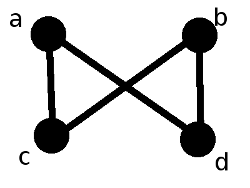# Join and meet

Let $$\langle P, \leq \rangle$$ be a partially ordered set, and let $$S \subseteq P$$. The join of $$S$$ in $$P$$, denoted by $$\bigvee_P S$$, is an element $$p \in P$$ satisfying the following two properties:

• p is an upper bound of $$S$$; that is, for all $$s \in S$$, $$s \leq p$$.

• For all upper bounds $$q$$ of $$S$$ in $$P$$, $$p \leq q$$.

$$\bigvee_P S$$ does not necessarily exist, but if it does then it is unique. The notation $$\bigvee S$$ is typically used instead of $$\bigvee_P S$$ when $$P$$ is clear from context. Joins are often called least upper bounds or supremums. For $$a, b$$ in $$P$$, the join of $$\{a,b\}$$ in $$P$$ is denoted by $$a \vee_P b$$, or $$a \vee b$$ when $$P$$ is clear from context.

The dual concept of the join is that of the meet. The meet of $$S$$ in $$P$$, denoted by $$\bigwedge_P S$$, is defined an element $$p \in P$$ satisfying.

• p is a lower bound of $$S$$; that is, for all $$s$$ in $$S$$, $$p \leq s$$.

• For all lower bounds $$q$$ of $$S$$ in $$P$$, $$q \leq p$$.

Meets are also called infimums, or greatest lower bounds. The notations $$\bigwedge S$$, $$p \wedge_P q$$, and $$p \wedge q$$ are all have meanings that are completely analogous to the aforementioned notations for joins.

## Basic exampleThe above Hasse diagram represents a poset with elements $$a$$, $$b$$, $$c$$, and $$d$$. $$\bigvee \{a,b\}$$ does not exist because the set $$\{a,b\}$$ has no upper bounds.$$\bigvee \{c,d\}$$ does not exist for a different reason: although $$\{c, d\}$$ has upper bounds $$a$$ and $$b$$, these upper bounds are incomparable, and so $$\{c, d\}$$ has no least upper bound. There do exist subsets of this poset which possess joins; for example, $$a \vee c = a$$, $$\bigvee \{b,c,d\} = b$$, and $$\bigvee \{c\} = c$$.

Now for some examples of meets.$$\bigwedge \{a, b, c, d\}$$ does not exist because $$c$$ and $$d$$ have no common lower bounds. However, $$\bigwedge \{a,b,d\} = d$$ and $$a \wedge c = c$$.